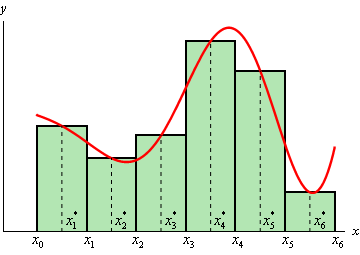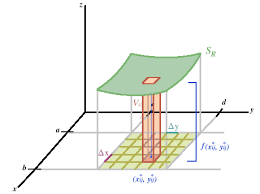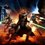# Guide to solving double integral using midpoint method for Neeraj

The midpoint rule works by choosing midpoint values of $x$ and finding the area under the curve by approximating using rectangles. An image below is shown to illustrate the idea:The higher dimension version of this is with double integrals, where the integral is approximated using rectangular prisms, where the rectangular prism's height is $f(\overline{x}, \overline{y})$, and the area of the base is $\Delta x \Delta y$.

Here's an image for some clarity on how it works:Thus the area of the individual prism is $f(\overline{x}, \overline{y})\Delta x \Delta y$. Here's the code:

  1 2 3 4 5 6 7 8 9 10 11 12 13 14 15 16 17 18 19 20 21 22 23 24 25 26 27 28 29 30 31 32 33 34 35 36 37 38 39 40 41 42 43 44 45 46 import math def multivariableFunction(p,q): #Multivariable function z = f(x,y) return (p**2)*(q**3) #returning x^2 y^3 n=2000 #accuracy/squareroot of number of prisms a=0 #bottom value of x integral b=1 #top value of x integral c=0 #bottom value of y integral d=2 #top value of y integral deltaX=(b-a)/n #dx (side length of prism cross section) deltaY=(d-c)/n #dy (side length of prism cross section) xValues=[a+(b-a)/(2*n)] #first midpoint for i in range(0,n-1): xValues.append(xValues+deltaX) #midpoint x values deltaX = deltaX + (b-a)/n #midpoint increment yValues=[c+(d-c)/(2*n)] #y values list for i in range(0,n-1): yValues.append(yValues+deltaY) #adding to y values list deltaY = deltaY + (d-c)/n #midpoint increment zValues=[] #z (height of prism) values for i in xValues: for j in yValues: zValues.append(multivariableFunction(i,j)) #passing in arguments x and y to the function; adding to z values deltaA=(b*d-b*c-a*d+a*c)/n**2 #area values doubleIntegralValue=sum(zValues) #addition of all heights doubleIntegralValue=doubleIntegralValue*deltaA #collective volume of rectangular prisms print('The definite double integral, evaluated by the midpoint rule is: '+ str(doubleIntegralValue)) 

What this code does is that it manually splits up the $x$ and $y$ domain we are integrating on into very small parts, and computes the volumes of the rectangular prisms that is resulted from that.

It gets the value of the midpoints, and finds the values of the functions at that point, and multiplies by the small cross-section area of the rectangular prism.

It later sums up all the values of the volumes to end up at the value of the double integral. Try the code out for yourself.

To point you out some resources, I learnt this from Stewart Calculus Early Transcendentals.Note by Krishna Karthik
1 week ago

This discussion board is a place to discuss our Daily Challenges and the math and science related to those challenges. Explanations are more than just a solution — they should explain the steps and thinking strategies that you used to obtain the solution. Comments should further the discussion of math and science.

When posting on Brilliant:

• Use the emojis to react to an explanation, whether you're congratulating a job well done , or just really confused .
• Ask specific questions about the challenge or the steps in somebody's explanation. Well-posed questions can add a lot to the discussion, but posting "I don't understand!" doesn't help anyone.
• Try to contribute something new to the discussion, whether it is an extension, generalization or other idea related to the challenge.

MarkdownAppears as
*italics* or _italics_ italics
**bold** or __bold__ bold
- bulleted- list
• bulleted
• list
1. numbered2. list
1. numbered
2. list
Note: you must add a full line of space before and after lists for them to show up correctly
paragraph 1paragraph 2

paragraph 1

paragraph 2

[example link](https://brilliant.org)example link
> This is a quote
This is a quote
    # I indented these lines
# 4 spaces, and now they show
# up as a code block.

print "hello world"
# I indented these lines
# 4 spaces, and now they show
# up as a code block.

print "hello world"
MathAppears as
Remember to wrap math in $$ ... $$ or $ ... $ to ensure proper formatting.
2 \times 3 $2 \times 3$
2^{34} $2^{34}$
a_{i-1} $a_{i-1}$
\frac{2}{3} $\frac{2}{3}$
\sqrt{2} $\sqrt{2}$
\sum_{i=1}^3 $\sum_{i=1}^3$
\sin \theta $\sin \theta$
\boxed{123} $\boxed{123}$

Sort by:

@Krishna Karthik sir i am getting wrong answer for this problem
$\int_{0}^{2} \int_{0}^{1} x^{2} y^{3} dxdy$

  1 2 3 4 5 6 7 8 9 10 11 12 13 14 15 16 17 18 19 20 21 22 23 24 25 26 27 28 29 30 31 32 33 34 35 36 37 38 39 40 41 42 43 44 45 46 47 48 import math def multivariableFunction(p,q): return (i**2)*(j**3) n=4000 a=0 b=1 c=0 d=2 deltaX=1/4000 deltaY=2/4000 xValues=[0+1/(2*4000)] for i in range(0,n-1): xValues.append(xValues+deltaX) deltaX = deltaX = 1/4000 yValues=[0+2/(2*4000)] for i in range(0,n-1): yValues.append(yValues+deltaY) deltaY = deltaY + 2/4000 zValues=[] for i in xValues: for j in yValues: zValues.append(multivariableFunction(i,j)) deltaA=2/(4000**2) doubleIntegralValue=sum(zValues) doubleIntegralVlue=doubleIntegralValue*deltaA print('The definite double integral,evaluated by the midpoint rule is:'+str(doubleIntegralValue)) #======================================= RESTART: E:\test.py.txt ====================================== #The definite double integral,evaluated by the midpoint rule is:4.498999859397043 #>>> " 

Don't have to call me sir bro, I am younger than you.

- 1 week ago

@Krishna Karthik as you are guiding me so much therefore .

Bro you're so humble and respectful.

- 1 week ago

I know where you copied the code wrong. In the function definition, you have used "i" and "j". Instead use letters "p" and "q" because those are the arguments the function uses.

- 1 week ago

@Krishna Karthik i have typed the whole code.
the above double integral have two variables x and y and like that the double integral which we were evaluating have p and q ??
am i right ??
after correcting that line as p and q ,still i am getting wrong answer .

I am getting the right answer; 1.33333

- 1 week ago

@Krishna Karthik share that code with me

No problem mate. I just used the code above. Don't type it. Copy-paste the code using ctrl+c and ctrl+v, or cmd+c and cmd+v.

- 1 week ago

@Krishna Karthik i was just typing the code beacause i want to increase my typing speed and to memorise the code

Ah, fair enough :) My typing speed kinda sucks lmao

- 1 week ago

- 1 week ago

@Krishna Karthik thanks i agree with you.
cheers!

The names p and q are arbitrary; the function definition simply uses p and q. The values x and y are used in the function in the for loop.

- 1 week ago

And don't hard code the values. I declared variables n, a, b, c, and d so that there is no hard coding. Hard coding leads to errors.

- 1 week ago

@Krishna Karthik what is the meaning of hard coding ??

It's when you use the values like "4000" when it is already declared as "n". For "xValues", you just wrote in the numbers. Use the variables declared up on the top.

- 1 week ago

@Krishna Karthik after copying the code still i am getting $0.888888$ as answer.

Did you get the correct answer? I edited the code above now and realised the error; use the code above :)

- 1 week ago

@Krishna Karthik now the current has come and i checked ,
now i am getting correct answer. thanks
btw can we solve 4 integrals also?
if yes 5 also ??
can you tell me your PC specs

Yes, we can keep iterating the process and extend it to all dimensions; although the visualisations would be impossible. We can solve all dimensions of integrals with this family of methods :)

I have two PCs that I use; one is a mac with Intel i7 processor, and my best PC is a Windows 10 gaming PC with i7 processor (2.9 Ghz) and GeForce GTX 1070 GPU. It has 32 GB RAM.

- 1 week ago

@Krishna Karthik i also like to play games.
Do you play PUBG(MOBILE)??, I play but very rarely.
If yes, we will play 1-2 matches.

- 6 days, 23 hours ago

I play PUBG on PC. I used to play mobile so much though! Sadly, I can only on my crappy phone :(

- 6 days, 23 hours ago

Bro do you play CS:GO?

- 6 days, 23 hours ago

@Krishna Karthik Sorry sir .

I only play one game and it's pubg twice a week, or even doesn't play.
Btw Can we play 1 match in mobile?

- 6 days, 23 hours ago

I don't have mobile tho, sorry. My mobile keeps crashing when I play it lol.

- 6 days, 23 hours ago

@Krishna Karthik can you guide me to download it in PC in best way.
In YouTube there are so many video and they all tell different ways to download, which gets very messy.

- 6 days, 23 hours ago

Just before you download PUBG PC, I just wanna say it costs 40 dollars in Australia, and that you require a really good PC to play it.

If you do have a beast PC, you should be able to play it. I think I'll download mobile. There's no need to download PC and spends 40 dollars lol

- 6 days, 20 hours ago

@Krishna Karthik yes sir i agree with you.

- 6 days, 20 hours ago

@Krishna Karthik here below my python code is attached ,i have copied the whole code

  1 2 3 4 5 6 7 8 9 10 11 12 13 14 15 16 17 18 19 20 21 22 23 24 25 26 27 28 29 30 31 32 33 34 35 36 37 38 39 40 41 42 43 44 45 46 47 48 49 import math def multivariableFunction(p,q): return (p**2)*(q**2) n=5000 a=0 b=1 c=0 d=2 deltaX=(b-a)/n deltaY=(d-c)/n xValues=[a+(b-a)/(2*n)] for i in range(0,n-1): xValues.append(xValues+deltaX) deltaX = deltaX + (b-a)/n yValues=[c+(d-c)/(2*n)] for i in range(0,n-1): yValues.append(yValues+deltaY) deltaY = deltaY + (d-c)/n zValues=[] for i in xValues: for j in yValues: zValues.append(multivariableFunction(i,j)) deltaA=(b*d-b*c-a*d+a*c)/n**2 doubleIntegralValue=sum(zValues) doubleIntegralValue=doubleIntegralValue*deltaA print('The definite double integral, evaluated by the midpoint rule is: '+ str(doubleIntegralValue)) #========================================== RESTART: E:\test.py.txt ========================================= #The definite double integral, evaluated by the midpoint rule is: 0.8888888711109454 #>>>" 

I have added the comments. Meanwhile I will figure out the error :)

- 1 week ago

Oh, I have figured out the error in my code; the function was copied as $x^2 y^2$, not $x^2 y^3$, it was my error, soz. Have a go with the new code above! :)

- 1 week ago

The code should be working perfectly now; I have modified the function with the right expression.

- 1 week ago

@Krishna Karthik sir can you make a guide that how to evaluate integrals using Simpson $1/3$ and $1/8$ rule.

- 5 days, 3 hours ago

Alright. I'll do it soon and show you.

- 5 days, 3 hours ago